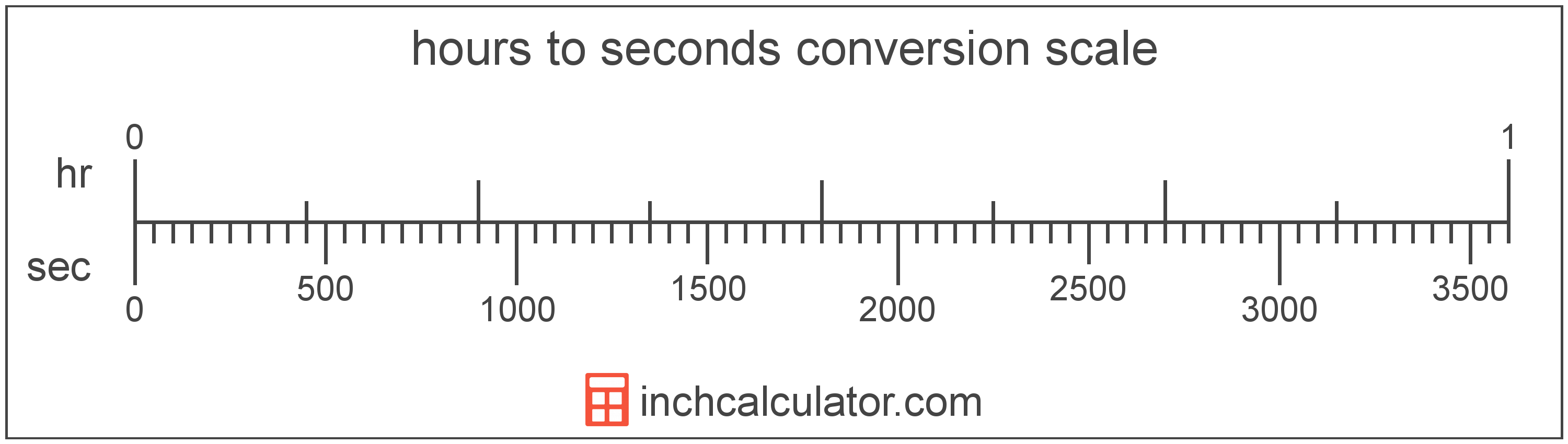# Seconds to Hours Converter

Enter the time in seconds below to get the value converted to hours.

Results in Hours:5,400 sec = 1.5 hrs
5,400 sec = 1 hr 30 min

Do you want to convert hours to seconds?

## How to Convert Seconds to Hours

The fastest way to convert seconds to hours is to use this formula:

hours = seconds ÷ 3,600

The time in hours is equal to the time in seconds divided by 3,600. Since there are 3,600 seconds in one hour, that's the conversion ratio used in the formula.

For example, here's how to convert 5,400 seconds to hours using the formula above.

hours = (5,400 sec ÷ 3,600) = 1.5 hr

### How to Convert Seconds to Hours, Minutes & Seconds

The formula above can be used to find the total hours in decimal form, but if you want to convert seconds into whole hours, minutes and seconds, then follow these steps.

• Divide the total seconds by 3,600 to get the total hours in decimal form. Then, use the number to the left of the decimal point as the number of whole hours.
• Find the remaining minutes by subtracting the whole hours from the total hours, then multiplying the result by 60. The number to the left of the decimal point is the number of whole minutes.
• Find the remaining seconds by subtracting the whole minutes from the remaining minutes found above, then multiplying the result by 60. The result is the number of seconds remaining.
• Finally, put the number of whole hours, minutes, and remaining seconds into the form HH:MM:SS where HH are the whole hours, MM are the whole minutes, and SS are the remaining seconds.## What is a Second?

The second has historically been defined as 1/60 of a minute or 1/86,400 of a day. According to the SI definition, the second is the duration of 9,192,631,770 oscillations of the frequency of light emitted from the atomic transition between the two hyperfine levels of the unperturbed ground state of the caesium 133 atom.

The second is the SI base unit for time in the metric system. Seconds can be abbreviated as s, and are also sometimes abbreviated as sec. For example, 1 second can be written as 1 s or 1 sec.

## What is an Hour?

The hour is a period of time equal to 1/24 of a day or 60 minutes.

The hour is an SI accepted unit for time for use with the metric system. Hours can be abbreviated as hr (plural hrs); for example, 1 hour can be written as 1 hr, and 2 hours can be written as 2 hrs.

## Second to Hour Conversion Table

Seconds converted to hours and hours, minutes & seconds.
Seconds Hours Hours, Minutes & Seconds
1 sec 0.000278 hr 0 hrs 0 min 1 sec
100 sec 0.027778 hr 0 hrs 1 min 40 sec
200 sec 0.055556 hr 0 hrs 3 min 20 sec
300 sec 0.083333 hr 0 hrs 5 min
400 sec 0.111111 hr 0 hrs 6 min 40 sec
500 sec 0.138889 hr 0 hrs 8 min 20 sec
600 sec 0.166667 hr 0 hrs 10 min
700 sec 0.194444 hr 0 hrs 11 min 40 sec
800 sec 0.222222 hr 0 hrs 13 min 20 sec
900 sec 0.25 hr 0 hrs 15 min
1,000 sec 0.277778 hr 0 hrs 16 min 40 sec
2,000 sec 0.555556 hr 0 hrs 33 min 20 sec
3,000 sec 0.833333 hr 0 hrs 50 min
3,600 sec 1 hr 1 hr
4,000 sec 1.1111 hr 1 hr 6 min 40 sec
5,000 sec 1.3889 hr 1 hr 23 min 20 sec
6,000 sec 1.6667 hr 1 hr 40 min
7,000 sec 1.9444 hr 1 hr 56 min 40 sec
8,000 sec 2.2222 hr 2 hrs 13 min 20 sec
9,000 sec 2.5 hr 2 hrs 30 min
10,000 sec 2.7778 hr 2 hrs 46 min 40 sec
20,000 sec 5.5556 hr 5 hrs 33 min 20 sec
30,000 sec 8.3333 hr 8 hrs 20 min
40,000 sec 11.11 hr 11 hrs 6 min 40 sec
50,000 sec 13.89 hr 13 hrs 53 min 20 sec
60,000 sec 16.67 hr 16 hrs 40 min
70,000 sec 19.44 hr 19 hrs 26 min 40 sec
80,000 sec 22.22 hr 22 hrs 13 min 20 sec
86,400 sec 24 hr 24 hrs
90,000 sec 25 hr 25 hrs
100,000 sec 27.78 hr 27 hrs 46 min 40 sec

## References

1. International Bureau of Weights and Measures, The International System of Units, 9th Edition, 2019, https://www.bipm.org/documents/20126/41483022/SI-Brochure-9-EN.pdf